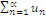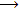# Explain the sequence of partial sums of an infinite series

Publish On: 2019-03-16

Total Post: 571

# Mjay Jollay

Total Post: 0

## ANS: Explain the sequence of partial sums of an infinite series

In order to give a meaning to the sum of an infinite series Σun, we form a sequence of partial sums.

Let= u1 + u2 + u3 + …. + un + …. be any given series, where the terms may be positive ir negative.

Let Sn = u1 + u2 + …. + un, be the sum of first n terms of the series Σun. Then Sn is called the partial sum of the first n terms of the given series and the sequence <Sn>, where Sn = u1 + u2 + …. + un, ∀ n ϵ N, is called the sequence of the partial sums of given series.

If Sn is known, we can find un and hence the series,

for un = Sn – Sn-1

∴ From the above discussion, we conclude that a sequence <Sn> of partial sums is associated to each seriesand vice versa.

∴ We can assign the same behaviour to the seriesas is exhibited by its sequence <Sn> of partial sums as n∞.